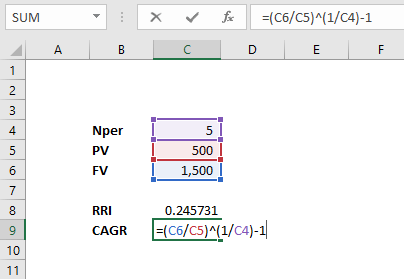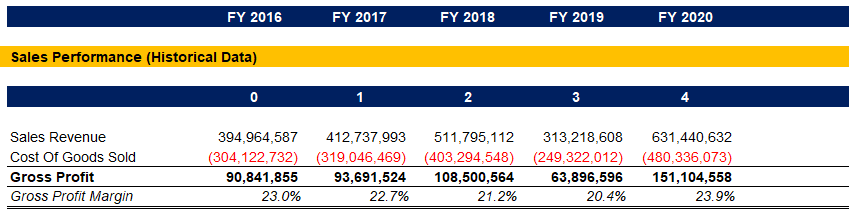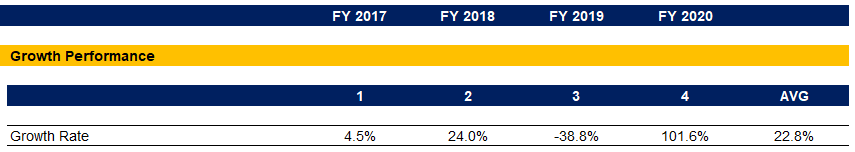## Introduction

Investors are continually evaluating different investments, trying to identify the ones that will maximize their returns and wealth. Analysts consider the Compound Annual Growth Rate (CAGR) as one of the most accurate ways to calculate the return for any investment with a value that changes over time.

CAGR represents the rate of return an investment would require, to grow from its beginning value to its future end value, with the underlying assumptions that we reinvest all profits over the period. The ratio is instrumental in investment analysis as it allows professionals to calculate a smoothed rate of return, which they can compare between varying investments.

## Compound Annual Growth Rate (CAGR)

The metric is not a ‘true’ rate of return. It is more of a representational one. CAGR is described as the rate at which an investment would’ve grown if it kept growing steadily over the years, and we reinvested all profits. In reality, this is highly unlikely.

In mathematical terms, CAGR is a geometric progression ratio that gives a constant rate of return over a defined period.

One of the shortcomings of CAGR is that it ‘hides’ volatility and investment risk by smoothing performance. To work, the metric implies that the growth rate during the investment’s life span is steady, which is rarely the case.

Instances, when investors add funds to their portfolio, provide another shortcoming for the Compound Annual Growth Rate. The measure does not account for such additional amounts and includes them in the returns instead, which inaccurately inflates CAGR.

### Applications

The Compound Annual Growth Rate (CAGR) helps us smooth returns over a more extended period, so we can easily compare investments. It’s particularly useful when the compared instruments show a highly volatile performance over the years.

We can use the metric to track the performance and development of any metric that changes value over time. Comparing the CAGR of different measures is a great way to reveal strengths and identify weaknesses within the company. We can also compare these to other companies to find our competitive advantage.

The Compound Annual Growth Rate is also helpful in financial planning and budgeting. We can use it to forecast future performance based on historical data by calculating the ratio and applying it for the next periods.

In some cases, the volatility in financial measures can render traditional horizontal analysis irrelevant. CAGR helps us mitigate the effect of this volatility by smoothing performance over the entire period. It is particularly suitable for comparing metrics between different companies in the same industry, such as revenue growth, EBITDA growth, and others.

### CAGR and Internal Rate of Return (IRR)

The Internal Rate of Return metric also calculates the rate of return. However, it is more flexible than CAGR, as we can apply it to more complex instruments with multiple periods and varying cash flows.

The Compound Annual Growth Rate takes into account one initial investment and one ending value to calculate the return. IRR incorporates multiple cash flows to calculate the return on investment. We use the Internal Rate of Return in more complex scenarios. An example can be a venture capital firm deciding which acquisition targets could provide the highest returns. Or a company is determining which of several optimization projects will result in a better return on investment.

### Compound Annual Growth Rate and Total Return

The Total Return metric is the most straight-forward rate of return. We calculate it with the formula:Where:

• FV is the investment’s future value; and
• PV is the investment’s present value.

The Total Return doesn’t take into account the time it took for the investment to accumulate its future value. The Compound Annual Growth Rate, on the other hand, takes into consideration the concept for the time value of money. This makes CAGR a better option when comparing instruments with different life spans.

## Calculating the Compound Annual Growth Rate

We calculate the Compound Annual Growth Rate as a hypothetical constant compounding interest rate, which will turn a given present value to its future value (also given).

We calculate it with the following formula:As CAGR is a ratio, we can use either actual or normalized data, as long as we keep the same mathematical proportions.

It is common to use the formula to calculate a hurdle rate of return. We can set a desired future value of our portfolio and calculate the required return on investment to achieve this value with the funds available now.

Investments are not usually made at the beginning of one year and released at the end. To accommodate this, we calculate fractions of years when applying the CAGR formula.

### Calculating in Excel

There is no CAGR function in Microsoft Excel. We can use the RRI function instead. The syntaxis is

`RRI(Nper, PV, FV)`

Where:

• Nper is the number of periods in the life span of the investment;
• PV is the present value; and
• FV is the future value.

We can also create the formula for CAGR in Excel, using the same values:

`= (FV/PV)^(1/Nper) - 1`

Setting this up in Excel would look like this and will yield the same result as the RRI function:## Example application of the Compound Annual Growth Rate

As with all our articles, we will take a look at a practical example to illustrate better how we can use the Compound Annual Growth Rate metric in our financial models.

We have the Sales performance of a company for the past five years.We have the objective to forecast the company’s sales performance for the next five years, as part of our financial modeling efforts.

One approach we might take is to calculate the year-on-year growth rate and then make an average of the results.

Doing so gives us an average growth rate of 23%.Another option is to calculate the growth with the Total Return ratio. We arrive at a growth rate of 15%. However, this doesn’t consider the fact that the time is longer than one period, and the significantly lower performance in the financial year 2019.Here the Compound Annual Growth Rate ratio comes as a better fit. By calculating CAGR, we consider the time value of money concept and the ‘losses’ generated in FY 2019 (meaning negative growth).

CAGR sits at 12%, and we can check our formula with the RRI function.Based on the information we can conclude our best option is to rely on the CAGR ratio for our forecast.

As you can see below, both the Average Growth and the Total Return ratios would have significantly overstated our revenue forecast.If you try the same exercise with a company showing steady sales growth, you will notice that the Average Growth ratio can also provide a reasonable forecast.

## Conclusion

CAGR is an excellent analytical tool that we can use to smooth out returns of investments, compare performance, forecast future development, and analyze metrics over a series of periods. It is the growth rate that will take a compounding investment from its present value to its future value. It is a better ratio than average annual return, as it considers losses.

Some financial analysts argue that the Compound Annual Growth Rate is not reality, but rather an academic concept. However, it is still a potent analytical tool over long periods, that allows us to compare the performance of investments and various financial metrics.

It is essential to remember that CAGR gives a ‘smoothed version’ of reality, and eliminating volatility can be dangerous in financial planning and modeling.

## And Get a FREE Benchmark Analysis Template## FCCA, FMVA

Hi! I am a finance professional with 10+ years of experience in audit, controlling, reporting, financial analysis and modeling. I am excited to delve deep into specifics of various industries, where I can identify the best solutions for clients I work with.

In my spare time, I am into skiing, hiking and running. I am also active on Instagram and YouTube, where I try different ways to express my creative side.